# A New Type-2 Fuzzy Sliding Mode Control for Longitudinal Aerodynamic Parameters of a Commercial Aircraft

A New Type-2 Fuzzy Sliding Mode Control for Longitudinal Aerodynamic Parameters of a Commercial Aircraft
Department of Electrical Engineering, Faculty of Engineering, Ilam University, Ilam 69311, Iran

Corresponding Author Email:
j.tavoosi@ilam.ac.ir
Page:
479-485
|
DOI:
https://doi.org/10.18280/jesa.530405
4 June 2020
|
Accepted:
1 August 2020
|
Published:
30 September 2020
| Citation

OPEN ACCESS

Abstract:

Although sliding mode control has many advantages such as stability and robustness but there are two important disadvantages as follow: Chattering phenomenon and mathematical nonlinear dynamic equivalent controller part. So, this paper presents a new method of adaptive sliding mode control based on general type-2 fuzzy logic to overcome on the mentioned problems. First, the longitudinal motion equations of a commercial aircraft and the upper limits of the unknown functions are introduced, which include the driving errors and uncertain parameters of the model. Then, a general type-2 fuzzy neural network (GT2FNNs), with adaptive rules, estimates these limits. Estimating the limits can reduce the computational load with less rules and weight than the dynamic matrix. The Boeing 747 is being studied and an attempt has been made to use a model very close to this aircraft. The stability of the control system has been proven. The simulation results show that by applying three models of faults to the aircraft system, the proposed type-2 fuzzy-based sliding mode control has excellent performance, especially in controlling the Aileron and Rudder angles.

Keywords:

general type-2 fuzzy, SMC, parameters uncertainty, Boeing 747

1. Introduction

The Boeing 747 is one of the most popular commercial jets in the world. Given the high demand for the aircraft and the growing number of flights, researchers are thinking of designing more accurate and reliable control systems for these aircraft. It is a large passenger aircraft and therefore has complex nonlinear systems and many variables . Flight parameters, including speed, altitude, and angle of attack, are constantly changing, leading to changes in aircraft dynamics. Unauthorized deviation of at least one of the variables may lead to a fault . The faults may cause some systems to malfunction, or even some physical equipment to break, or eventually cause the aircraft to crash . Therefore, it is necessary to quickly identify the faults and compensate them. This operation is one of the tasks of the control system. After detecting the faults, Fault-Tolerant Flight Control Systems (FTFCS) take the aircraft to the autopilot control mode and prevent the aircraft from crashing . There are several ways to control a commercial plane, some of which are discussed below. The fractional order method is used to surface control of a commercial aircraft by compensating with PI controller . In the mentioned paper, an aerial load is applied to challenge the control system, but the parameters have not any uncertainty. A model reference adaptive control (MRAC)-based SMC has been proposed for the Boeing 747 . The MRAC is basically depends on exact mathematical model of the aircraft, therefore if this model differs from the aircraft model, the controller will not work well. The MRAC-based SMC has been used for control of longitudinal motion of a Boeing 747 aircraft . In the mentioned paper, the adaptation law is derived from Lyapunov stability theory, however, the linear model is still considered (MRAC) and the parameter uncertainty is not considered. To prevent multiple switching , a controller approach based on the H2 multivariate control / regulator theory is presented to control the aircraft. A control method based on feedback linearization design for aircraft multi-variable nonlinear dynamics with unknown excitation faults and unknown disturbances has been presented . In the mentioned paper, a direct adaptive controller is generated to monitor the faults from parameters uncertainty, and then an integrated comparator with adaptive weight can provide effective compensation for the effect on the faults. Complexity and difficult implementation are the disadvantages of the presented method in the paper . Unfortunately, most of the above methods have used the linear mathematical model of the aircraft to design the controller, while the nature of the dynamic behavior of flight systems is nonlinear. The difference between a linear model and a non-linear model is sometimes huge and unavoidable. So, we have to fill this gap somehow. One way is to use tools based on computational intelligence.

Computational intelligence tools in complex systems have performed well in terms of modeling and system identification [10-13], control and regulation [14-17], and so on [18-20]. Neural networks, fuzzy logic, and evolutionary algorithms have been very efficient in combining model-based methods (control theory) in aerospace systems [21, 22]. A neural network with lyapunov-based adaptation law is used to robust control of an airplane . The tracking control scheme with a radial base function neural network compensator for a commercial aircraft has been proposed . In the mentioned paper, the saturation of the actuators and the uncertainty of the aerodynamic parameters are considered. The uncertainty of aerodynamic parameters is online regulated by neural networks and allocation control law. A sliding mode control (SMC)-based fault tolerant for aircraft system control has been presented . In the mentioned paper, a neural network is used to detect faults and eliminate them with the Lyapunov -based learning algorithm. The disadvantages of the proposed method in the paper  are that the equations are very general and there are no restrictions. A control system consists of three internal loops has been proposed . In the mentioned paper, the inner ring is responsible for controlling the actuation force by a PI controller, the middle ring controls the position of the body by a fuzzy controller similar to PD, and the outer ring is the impedance control ring. A type-1 fuzzy sliding mode control has been used as fault-tolerant control of a commercial aircraft . In the papers reviewed above, either only one fault factor is considered (several sources of fault have not been analyzed) or the difference between the linearized model and the nonlinear model has not been compensated, therefore, present paper has been written to eliminate these problems. Another innovation of this paper is reduction the effect of chattering phenomenon, which is one of the challenges of the sliding model control method, by using general type-2 fuzzy logic as soft switching.

In this paper firstly a mathematical model to describe the longitudinal behavior of a commercial aircraft is presented. Then the general type-2 fuzzy system is expressed. In the following, the sliding mode adaptive control method based on the general type-2 fuzzy system is presented and finally simulation and conclusion are expressed.

2. The Longitudinal Model of the Aircraft

In aircraft dynamics, although the analysis and design approaches in this study are not limited to the specific type of aircraft, it is better to pay attention to a special aircraft system to explain the concepts and validate the design process. The Boeing 747 Series 200/100 is used as one of the most famous and widely used commercial jet aircraft, for example, in this research to explain the design process of SMC. The longitudinal motion of the Boeing 747's body axes can be presented as follows , regardless of its flexible effects:

$\dot{q}=\dot{C}_{7} M_{y}$      (1)

$\dot{V}=\frac{F_{X} \cos \dot\alpha+F_{Z} \sin \alpha}{m}$     (2)

where, q is dynamic pressure, C7 is inertia coefficient, $\alpha$ is angle of attack, V is airspeed and m are aircraft mass. The dynamic axis-body forces and torques are explained as follows:

$F_{x}=-\bar{q} S_{r}\left(C_{D} \cos \alpha-C_{L} \sin \alpha\right)+T-m g \sin \theta$      (3)

F_{Z}=-\bar{q} S_{r}\left(C_{D} \sin \alpha+C_{L} \cos \alpha\right)-0.0436 T-$$m q \cos \theta (4) M_{y}=\bar{q} S_{r} \bar{C}\left[C_{m}-\frac{1}{\bar{C}}\left(C_{D} \sin \alpha+C_{L} \cos \alpha\right) \overline{x_{c g}}\right. \\ -\frac{1}{\bar{C}}\left(C_{D} \cos \alpha-C_{L} \sin \alpha\right) \overline{z_{c g}}+\frac{\overline{c \dot{\alpha}}}{V}\left(C_{m_{\alpha}}\right. \\ \left.\left.-\frac{\bar{x}_{c g}}{\bar{C}} C_{L_{\alpha}} \cos \alpha\right)\right]+Z_{e n g} T (5) where, Sr is reference surface area, CD, CL and Cm are the drag, the lift and pitching moment coefficients, respectively. The trust force is shown by T, xcg is center of gravity in x-axis and Zeng is engine position in z-axis. The aerodynamic coefficients for longitudinal motion can be expressed as follows: C_{L}=C_{L 0}+\frac{\bar{c}}{2 V}\left(1.45-1.8 \bar{x}_{C g}\right) \frac{d C_{L}}{d q} q \quad+K_{\alpha}\left(\frac{d C_{L}}{d \delta_{t e}} \delta_{i e}+\frac{d C_{L}}{d \delta_{o e}} \delta_{o e}\right) (6) C_{D}=C_{D_{M a c h}} (7) C_{m}=C_{m 0}+\frac{\bar{c}}{2 V} \frac{d C_{m}}{d q} q+K_{\alpha} \frac{d C_{m}}{d \delta_{s}} \delta_{s} +K_{\alpha}\left(\frac{d C_{m}}{d \delta_{t e}} \delta_{i e}+\frac{d C_{m}}{d \delta_{o e}} \delta_{o e}\right) (8) where, the inner elevator deflection and outer elevator deflection are shown by \delta_{i e} and \delta_{o e}, respectively. C_{D_{M a c h}} drag coefficient at a fixed Mach number and K_{\alpha} effective factor of the elevator. In addition, aerodynamic coefficients can be as follows: C_{D_{\text {Mach}}}=k_{20} \alpha^{2}+k_{10} V+k_{01} V+k_{00} (9) \frac{d c_{L}}{d \delta_{t e}}=\frac{d c_{L}}{d \delta_{o e}}=\tau_{02} V^{2}+\tau_{01} V+\tau_{00} (10) C_{L O}=\eta_{10} \alpha+\eta_{01} V+\eta_{00} (11) C_{m o}=\xi_{20} \alpha^{2}+\xi_{10} \alpha+\xi_{01} V+\xi_{00} (12) \frac{d C_{m}}{d \delta_{t e}}=\frac{d C_{m}}{D \delta_{o e}}=\zeta_{02} V^{2}+\zeta_{01} V+\zeta_{00} (13) where, in the paper : \left\{\begin{array}{l}k_{20}=3.67, K_{10}=3.48 \times 10^{-2}, K_{01}=4.45 \times 10^{-5}, K_{00}=9.92 \times 10^{-3} \\ \tau_{02}=-0.72 \times 10^{-7}, \tau_{01}=2.13 \times 10^{-5}, \tau_{00}=1.61 \times 10^{-3} \\ \eta_{10}=5.15, \eta_{01}=1.21 \times 10^{-3}, \eta_{00}=6.15 \times 10^{-3} \\ \xi_{20}=2.39, \xi_{10}=-1.46, \xi_{01}=-3.20 \times 10^{-4}, \xi_{00}=0.12 \\ \zeta_{02}=2.18 \times 10^{-7}, \zeta_{01}=-0.58 \times 10^{-4}, \zeta_{00}=0.88 \times 10^{-2}\end{array}\right. (14) Note 1: It is clear from Eq. (6) that the CL increase coefficient is based on the acute effects of peak angles, lift deviations, and the basic CLO component, respectively. Relationship (7) shows that the traction coefficient of CD is largely based on the effect of Mach number. As can be seen from Eq. (8), the fundamental factors affecting the Cm transverse torque coefficient actually include the peak angular velocity, the internal elevation deviation, the external elevation deviation, the stabilizing deviation, and the basic Cmo component, respectively. By substituting relationships (3)-(8) for relationships (1) - (3) we get that: q=\frac{\dot{C_{7}} \bar{q} S_{r} \bar{C}}{2 V}\left[\bar{C} \frac{d c_{m}}{d q}-\left(1.45-1.8 \bar{x}_{c g}\right)\left(\bar{x}_{c g} \cos \alpha+\bar{z}_{c g} \sin \alpha\right)\right] q +c_{7} \bar{q} S_{r} \bar{c} C_{m o}+c_{7} \bar{q} S_{r}\left[C_{D_{M a c h}}\left(\bar{z}_{c g} \cos \alpha-\bar{x}_{c g} \sin \alpha\right)\right. \left.-C_{L D}\left(\bar{x}_{c, g} \cos \alpha+\bar{z}_{c g} \sin \alpha\right)\right]$$+c_{7} \bar{q} S_{r} K_{\alpha}\left[\bar{c} \frac{d c_{m}}{d \delta_{L e}}-\left(x_{c g} \cos \alpha+\bar{z}_{c g} \sin \alpha\right) \bar{c} \frac{d c_{L}}{d \delta_{L e}}\right] \delta_{i e}+c_{7} \bar{q} S_{r} K_{\alpha}\left[\bar{c} \frac{d c_{m}}{d \delta_{o e}}-\left(\bar{x}_{c g} \cos \alpha+\bar{z}_{c g} \sin \alpha\right) \bar{c} \frac{d c_{L}}{d \delta_{o e}}\right] \delta_{o e} +c_{7} \bar{q} S_{r} K_{\alpha} \bar{c} \frac{d c_{m}}{d \delta_{s}} \delta_{s}+c_{7} z_{e n g} T (15) \dot{V}=-g \sin \gamma-\frac{\bar{q} s_{r}}{m} C_{D_{\text {Mach}}} +\frac{4(\cos \alpha-0.0436 \sin \alpha)}{m} (16) The parameters of aircraft cannot be accurately obtained, this leads to challenges in designing flight control. Usually, there are incremental parameters ( \Delta_{*} ) in nominal values: \left\{\begin{array}{l}m=m_{0}\left(1+\Delta_{m}\right) \\ s_{r}=s_{r o}\left(1+\Delta_{s_{r}}\right) \\ I_{y y=I_{y y o}}\left(1+\Delta_{I_{y y}}\right) \\ \bar{c}=\bar{c}_{o}\left(1+\Delta_{\xi}\right) \\ \bar{q}=\bar{q}_{o}\left(1+\Delta_{\bar{q}}\right)\end{array}\right. (17) If we define \mathrm{x}=[\mathrm{q}, \mathrm{V}]^{\mathrm{T}} , as well \mathrm{u}=\left[\delta_{\mathrm{ie}}, \delta_{\mathrm{oe}}, \delta_{\mathrm{s}}\right]^{\mathrm{T}} , the equations of longitudinal motion can be facilitated as follows: \begin{aligned} \dot{x}=f(x)+g(x) & u \\ &=\left(f_{o}(x)+\Delta_{f}\right) \\ &+\left(g_{o}(x)+\Delta_{g}\right) u \end{aligned} (18) where, f(x) \in R^{2}, g(x) \in R^{2 \times 3} are nonlinear functions of x. \mathrm{f}_{0}(\mathrm{x}) and \mathrm{g}_{0}(\mathrm{x}) represent the nominal phrases f(x), g(x), while \Delta_{\mathrm{f}}, \Delta_{\mathrm{g}} on uncertainty phrases (modeling errors / parameters uncertainties) related to f(x), g(x) respectively. Therefore, the phrase corresponding to the longitudinal motion of the aircraft can be represented by the relation (18) by calculating the impulse defects as follows: \dot{x}=\left(f_{o}(x)+\Delta_{f}\right)+\left(g_{o}(x)+\Delta_{g}\right)(\Lambda u+\tau) (19) Assumption 1: Suppose that the following inequalities are concluded: \left\|\Delta_{f}+\left(g_{o}+\Delta_{g}\right) \tau\right\| \leq r_{1} \quad \dot{q}=\dot{C}_{7} M_{y} (20) \left\|g_{o}(\Lambda-I) g_{o}^{+}+\Delta_{g} \Lambda g_{o}^{+}\right\| \leq r_{2}<1 (21) where, \mathrm{g}_{0}^{+} is the pseudo inverse of go and r1, r2 are the unknown positive parameters. 3. General Type-2 Fuzzy Neural Network The ability to learn neural networks is used to regulate the shape of fuzzy membership functions and output weights. In this paper, the purposed GT2FNN is to provide online upper limits (p1, p2) as a criterion for unknown expressions. It should be noted that the estimation process p1, p2 is variable from every time. A general type-2 fuzzy sets that used in this paper is shown in Figure 1. ## 1.pngFigure 1. General type-2 fuzzy set The mathematical definition of a general type-2 fuzzy set is as follows: \tilde{\tilde{A}}=\left\{\left((x, u), \mu_{\tilde{A}}(x, u) \mid \forall x \in X, \forall u \in J_{x} \subseteq[0,1]\right)\right\} (22) where, x is input, u is primary membership function and \mu_{\tilde{A}}(x, u) is secondary membership function. Note that J_{x} is an interval set as: J_{x}=\left\{(x, u): u \in\left[\underline{\mu}_{\tilde{A}}, \bar{\mu}_{\tilde{A}}\right]\right\}. A triangular interval type-2 fuzzy can be showed as: \tilde{A}=\left(\left\lceil\underline{l}_{A}, \bar{l}_{A}\right], m_{A},\left[\underline{r}_{A}, \bar{r}_{A}\right]\right). The upper and lower membership function are as: Upper \mu_{\tilde{A}}(x, u) =\left\{\begin{array}{cc}\frac{x-\underline{l}_{A}}{m_{A}-l_{A}} & \underline{l}_{A}<x<m_{A} \\ 1 & x=m_{A} \quad \dot{q}=\dot{C}_{7} M_{y} \\ \frac{x-\bar{r}_{A}}{m_{A}-\bar{r}_{A}} & m_{A}<x<\bar{r}_{A}\end{array}\right. (23) Lower \mu_{\tilde{A}}(x, u)=\left\{\begin{array}{ll}\frac{x-\bar{l}_{A}}{m_{A}-\bar{l}_{A}} & \bar{l}_{A}<x<m_{A} \\ 1 & x=m_{A} \\ \frac{x-\underline{r}_{A}}{m_{A}-\underline{r}_{A}} & m_{A}<x<\underline{r}_{A}\end{array}\right. (24) For simplicity we show \tilde{\tilde{A}} as A. A fuzzy rule is as follows: if x_{i} is A_{i}^{l}, THEN \rho_{1}(x)=W_{l} (25) where, \mathrm{i}=1,2, \ldots, \mathrm{m}, \mathrm{l}=1,2, \ldots, \mathrm{N}, \quad \rho_{1}=\mathrm{U}_{\mathrm{u}} \in \mathrm{R}^{\mathrm{m}} \rightarrow \mathrm{R}and \mathrm{A}_{\mathrm{i}}^{\mathrm{l}} indicates the fuzzy membership value of the input variable i in the l rule, and Wl is the output power associated with the l rule. The type 2 fuzzy neural network shown in Figure 2 consists of 4 layers. ## 2.png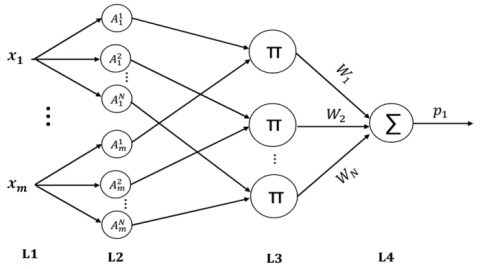Figure 2. Structure of type-2 fuzzy neural network Layer (1) receives the input variables. The membership values in layer (2) are calculated in such a way that the degree to which the input value is coordinated with a fuzzy set can be determined. Prerequisite coordination is done in layer (3). Prerequisites for fuzzy rules are identified by the links before layer (3), while the results are explained by the following links. Layer (4) is considered as the output layer. Therefore, the output of GT2FNN with N fuzzy rule is explained by Eq. (26): r_{1}(x)=W^{T} \tilde{\tilde{A}}\left\{\left((x, u), \mu_{\tilde{A}}(x, u) \mid \forall x \in X, \forall u \in J_{x}\right.\right.\subseteq[0,1])\}$$=\bar{W}^{T} \tilde{\tilde{A}}\left\{\left((x, u), \mu_{\tilde{A}}(x, u) \mid \forall x \in X, \forall u \in J_{x} \subseteq[0,1]\right)\right\}$$+\varepsilon_{1}      (26)

where, $\varepsilon_{1}$ is approximation error that It should be less and less and tend to be zero. Using GT2FNN, $\rho_{2}$ can be approximated in a manner similar to that described for $\rho_{1}$.

4. Sliding Mode Control Design Based on GT2FNN

As shown in Figure 3, the control system described here is essentially GT2FNN and SMC.

## 3.png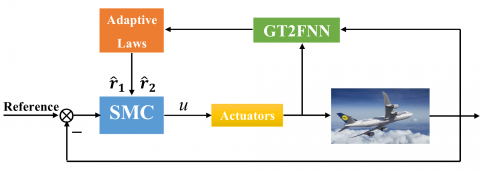Figure 3. Proposed control system structure

In the proposed method, GT2FNN has adaptive techniques used to obtain online values of $\hat{\mathrm{r}}_{1}, \hat{\mathrm{r}}_{2}$ . In this proposed structure, the role of GT2FNN is very important because it must be able to calculate the parameters $\hat{\mathrm{r}}_{1}, \hat{\mathrm{r}}_{2}$ online and by estimation. So we've tried to design a very good approximator so that it has good accuracy and low calculation time.

The vector defines the error as e=x-xd in which xd represents the command vector. In order to access the sliding mode in the whole response of the general system, the sliding surface is created as follows:

$s=e+\mu \int_{0}^{t}\left(\|e\|^{r} e /\|e\|\right) d \tau$     (27)

That μ is a positive constant and is $0<\mathrm{r}<1$. The purpose of the sliding mode control mentioned in this paper is to guide the tracking error vector towards the origin during S = 0 in a limited time under the faults and model uncertainties. According to the defective model of the aircraft in relation to (20), the SMC law is created as follows:

$u=-g_{0}^{+}\left[F+\left(\hat{r}_{1}+\hat{r}_{2}\|F\|+\eta\right) \frac{s}{\left\|_{S}\right\|}\right]$     (28)

wherein,

$F=f_{0}-\dot{x}_{d}+\mu\left(\frac{\|e\|^{r} e}{\|e\|}\right)$

$\eta \geq \frac{\left(\hat{r}_{1} \hat{r}_{2}+\hat{r}_{1} \tau_{2}^{0}+\tau_{1}^{0}\right)+\left(\hat{r}_{2} r_{2}+\hat{r}_{2} \tau_{2}^{0}+\tau_{2}^{0}\right)\|F\|}{1-\partial_{2}-\tau_{2}^{0}}+\varepsilon_{\eta}$

$\hat{r}_{1}=\widehat{W}^{T} \hat{\phi}, r_{2}=\hat{X}^{T} \hat{\psi}$

$\varepsilon_{\eta}>0$

$\hat{W}=\hat{\phi}$     (29)

$\dot{\hat{c}}_{l, i}=\phi_{i_{c_{l}}} \widehat{W}_{l}^{T}$    (30)

$\dot{\hat{\sigma}}_{l, i}=\phi_{i_{\sigma_{l}}} \widehat{W}_{l}^{T}$     (31)

$\dot{\hat{X}}=\hat{\rho}_{1} \hat{\psi}+\hat{\psi}\|F\|+\hat{\rho}_{2} \hat{\psi}\|F\|+\eta \hat{\psi}$     (32)

$\dot{\hat{p}}_{l, i}=\psi_{i, P_{l}} \hat{X}_{l}^{T}\|F\|+\hat{\rho}_{1} \psi_{i, P_{l}} \hat{X}_{l}^{T}+2 \psi_{i, P_{l}} \hat{X}_{l}^{T}\|F\|$

$+\eta \psi_{i, P_{l}} \hat{X}_{l}^{T}$     (33)

$\dot{\hat{q}}_{l, i}=\psi_{i, q_{l}} \hat{X}_{l}^{T}\|F\|+\hat{\rho}_{1} \psi_{i, q_{l}} \hat{X}_{l}^{T}+2 \psi_{i, q_{l}} \hat{X}_{l}^{T}\|F\|$

$+\eta \psi_{i, q_{l}} \hat{X}_{l}^{T}$     (34)

where, $\mathrm{i}=1,2, \ldots, \mathrm{m}, \quad \mathrm{l}=1,2, \ldots, \mathrm{N}$, therefore, the sliding mode control-designed rule ensures that the tracking error e can be traced to the origin during S = 0 in a limited time, even if there is an error in the operators and uncertainty in the model.

5. Simulation

The following are the automatic flight conditions:

$\alpha_{\text {trim }}=0.752^{\circ}, \mathrm{q}_{\text {trim }}=0, \mathrm{V}_{\text {trim }}=210 \mathrm{m} / \mathrm{s}, \mathrm{h}_{\text {trim }}=$

$6000 \mathrm{m}, \theta_{\text {trim }}=0.740^{\circ}, \delta_{\text {e }, \text { trim }}=0.650^{\circ}, \mathrm{T}_{\text {trim }}=40000 \mathrm{N}$

$\mu=10, \quad \eta=3$

Factors including model uncertainties, drive defects, and the size of measurement channels in simulations are considered.

• Factor 1: The mass of the aircraft is disturbed by up to 15% of its nominal value. $\mathrm{I}_{\mathrm{yy}}$ confusion is actually 15% of the nominal value. There is a maximum of 15% mismatch in $\overline{\mathrm{c}}, \overline{\mathrm{q}}, \mathrm{s}_{\mathrm{r}}$
• Factor 2: The faults of gain and bias for the thrust and the elevator are as follows:

$\lambda_{1}=\left\{\begin{array}{l}1,0 \leq t<7 \\ 0, \quad t \geq 7\end{array}, \sigma_{1}=\left\{\begin{array}{lr}1, & 0 \leq t<7 \\ 6, & t \geq 7\end{array}\right.\right.$      (35)

$\lambda_{2}=\left\{\begin{array}{c}1,0 \leq t<7 \\ 0.5, \quad t \geq 7\end{array}, \quad \sigma_{2}=\left\{\begin{array}{cc}1, & 0 \leq t<7 \\ 2, & t \geq 7\end{array}\right.\right.$     (36)

• Factor 3: The white noise is injected into each of the measuring channels with an average of zero and a coefficient of 0.01. In addition, in order to evaluate quantitative tracking performance, we define.:

$\left\{\begin{array}{l}e_{\theta}=\sqrt{\frac{1}{t_{1}-t_{0}} \int_{t_{0}}^{t_{1}}\left|\theta-\theta_{d}\right|^{2} d \tau} \\ e_{V}=\sqrt{\frac{1}{t_{1}-t_{0}} \int_{t_{0}}^{t_{1}}\left|V-V_{d}\right|^{2} d \tau}\end{array}\right.$    (37)

where, [t0, t1] covers the time frame of the general simulation. $\theta_{d}, V_{d}$ indicate the reference angles of altitude and velocity, respectively. For comparison, three control methods of general type-2 fuzzy neural network (T2FNN), type-1 fuzzy neural network-based control (T1FNN) and traditional sliding mode control (SMC) in simulations have been investigated. Figure 3 shows the control of the roll angle, in which three all three control systems can ensure the safety of the aircraft in the event of driving defects and model uncertainties. Closed loop behavior remains satisfactory, although error tracking displays a slightly worse transient behavior. As can be seen in Figure 4, the T2FNN-based SMC has a better response than other SMC designs. The root means square error (RMSE) in Figure 3 for T2FNN-based SMC, T1FNN-based SMC and alone SMC are 0.144, 0.387, and 0.435, respectively.

## 4.png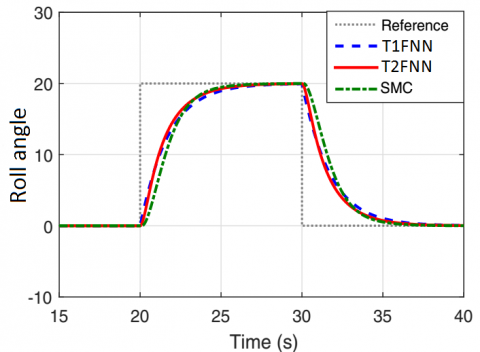Figure 4. Roll angle control in three ways

## 5.png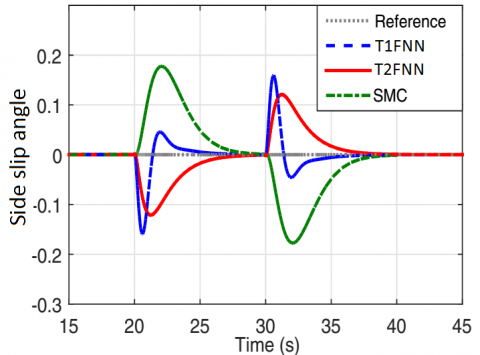Figure 5. The changes in the side slip angle with all three methods

Figure 5 shows the changes in the side slip angle with all three methods.

Side slip angle changes during flight indicate aircraft vibrations (left and right), so the smaller the angle changes, the more comfortable passengers will be. As can be seen in Figure 4, in the T2FNN-based SMC method, the angle changes softer and less. Figure 6 shows the control angle of the roll and the maximum faults due to uncertainty of the model and of the parameters and finally the faults of the operators (factors 1,2 and 3), with all three control methods.

As can be seen in Figure 5, the best performance among all three control methods is related to the T2FNN method. Figure 7 shows the Side slip angle changes with all three methods and with maximum faults (all three factors 1, 2 and 3).

## 6.png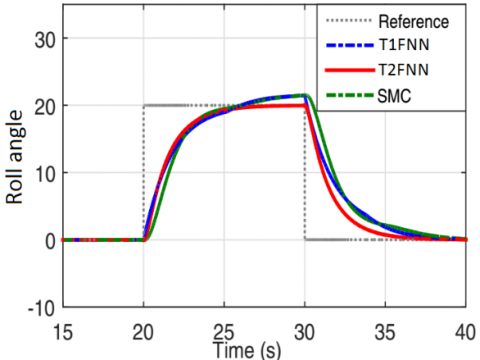Figure 6. Roll angle control by three methods and with maximum faults

## 7.png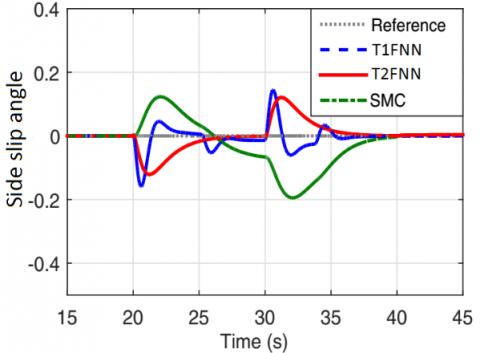Figure 7. Side slip angle changes with all three control methods and maximum faults

## 8.pngFigure 8. The aileron angle position for the T2FNN-based SMC

## 9.pngFigure 9. The position of the rudder angle for the T2FNN-based SMC

## 10.png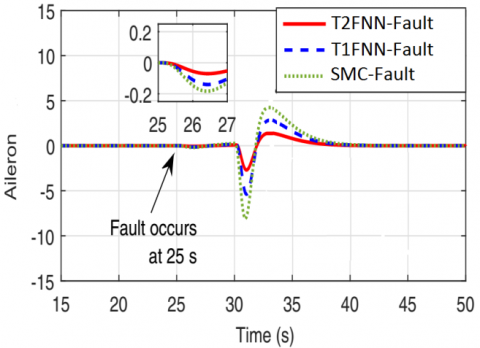Figure 10. Performance of all three control systems on the aileron angle by applying a fault

## 11.png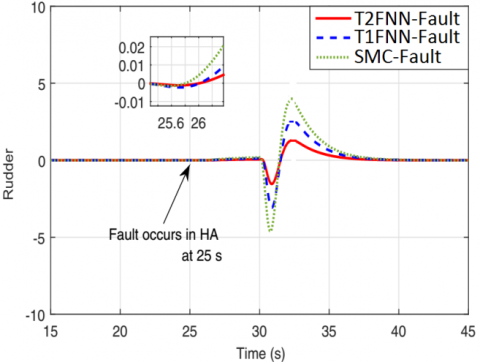Figure 11. The performance of all three control systems on the rudder angle by applying a fault

The aileron angle position for the T2FNN controller form Figure 4 is shown in Figure 8.

The position of the rudder angle for the T2FNN-based SMC for Figure 4 is shown in Figure 9.

We now consider the state of the aileron and rudder angles by applying a fault and comparing the performance of all three control methods. Suppose that at time t = 25s an actuator fault occurs in the system. Figure 10 shows the performance of all three control systems on the aileron angle by applying the fault.

As can be seen in Figure 10, a fault occurs in t = 25s after two seconds, i.e. in t = 27s, when a shake enters the aircraft it causes the aileron actuator to react. Figure 10 show that the best response is for T2FNN, as it has the least changes and overshoots, and quickly restores the system to its original state. Figure 11 shows the performance of all three control systems on the rudder angle by applying a fault.

At the rudder angle, which is responsible for directing the left and right in the direction of the horizon, as can be seen in Figure 11. A fault occurs in t = 25s after two seconds, i.e. in t = 27s, when a shake enters the aircraft it causes the rudder actuator to react. Due to the fact that the rudder has direct relation with the side slip angle control and the desired side slip angle is zero, the rudder should try to keep its position in presence of the inserted fault. Figure 11 show that the best response is for T2FNN, as it has the least changes and overshoots, and quickly restores the system to its original state.

6. Conclusions

In this paper, a new method of sliding mode control (SMC) based on the general type-2 fuzzy system for the longitudinal parameters of the Boeing 747 aircraft is presented. Faults due to parameters uncertainty, actuators, and noise can challenged the control system. The reaction of the control system may cause shocks on the plane and upset the passengers. Therefore, the control system must respond to the fault and make the least shocks to the aircraft and passengers while reacting in a quickly manner. The proposed T2FNN-based SMC has all the features mentioned above. Comparison of the proposed method with type-1 fuzzy-based SMC as well as the typical sliding model control (SMC) shows the superiority and efficiency of the proposed method. In this paper, we investigated the faults caused by the actuators, which for future research can also consider and analyze the faults caused by the sensors. We will also discuss the convergence and stability of the proposed control system in the next paper.

References

 Winter, S.R., Thropp, J.E., Rice, S. (2019). What factors predict a consumer's support of environmental sustainability in aviation? A multi-model analysis. International Journal of Sustainable Aviation, 5(3): 190-204. https://doi.org/10.1504/IJSA.2019.103502

 Qu, C.G., Cao, H.L., Sun, S., Xu, M.J. (2019). Modelling of fuel flow in climb phase through multiple linear regression based on the data collected by quick access recorder. Journal Européen des Systèmes Automatisés, 52(4): 409-413. https://doi.org/10.18280/jesa.520411

 Al-Ma'aiteh, T.I., Krammer, O. (2019). Thermoelectric generators simulation in aircraft applications. International Journal of Sustainable Aviation, 5(4): 313-323. https://doi.org/10.1504/IJSA.2019.105243

 Fadel, M.Z., Rabie, M.G., Youssef, A.M. (2019). Modeling, simulation and control of a fly-by-wire flight control system using classical PID and modified PI-D controllers. Journal Européen des Systèmes Automatisés, 52(3): 267-276. https://doi.org/10.18280/jesa.520307

 Salman, I., Lin, Y., Hamayun, M.T. (2018). Fractional order modeling and control of dissimilar redundant actuating system used in large passenger aircraft. Chinese Journal of Aeronautics, 31(5): 1141-1152. https://doi.org/10.1016/j.cja.2018.03.002

 Xiang, J., Zhang, S., Liu, Y., Ren, Z. (2018). Adaptive strategy for commercial aircraft with OFC fault. IEEE CSAA Guidance, Navigation and Control Conference (CGNCC), Xiamen, China, pp. 1-5. https://doi.org/10.1109/GNCC42960.2018.9019149

 Liu, Y., Dong, X., Ren, Z., Cooper, J. (2019). Fault-tolerant control for commercial aircraft with actuator faults and constraints. Journal of the Franklin Institute, 356(7): 3849-3868. https://doi.org/10.1016/j.jfranklin.2018.11.043

 Chan, P.C., Chang, B.C., Bayram, M., Kwatny, H., Belcastro, C.M. (2019). Robust tracking control of an aircraft with critical actuator jam failures. Asian Journal of Control, 1-19. https://doi.org/10.1002/asjc.2280

 Yao, X., Yang, W. (2020). Adaptive fault compensation and disturbance suppression design for nonlinear systems with an aircraft control application. International Journal of Aerospace Engineering, 2020: 1-16. https://doi.org/10.1155/2020/4531302

 Tavoosi, J., Suratgar, A.A., Menhaj, M.B. (2016). Nonlinear system identification based on a self-organizing type-2 fuzzy RBFN. Engineering Applications of Artificial Intelligence, 54: 26-38. https://doi.org/10.1016/j.engappai.2016.04.006

 Tavoosi, J., Suratgar, A.A., Menhaj, M.B. (2016). Stable ANFIS2 for nonlinear system identification. Neurocomputing, 182: 235-246. https://doi.org/10.1016/j.neucom.2015.12.030

 Tavoosi, J., Badamchizadeh, M.A. (2013). A class of type-2 fuzzy neural networks for nonlinear dynamical system identification. Neural Computing and Application, 23: 707-717. https://doi.org/10.1007/s00521-012-0981-7

 Tavoosi, J., Jokandan, A.S., Daneshwar, M.A. (2012). A new method for position control of a 2-dof robot arm using neuro-fuzzy controller. Indian Journal of Science and Technology, 5(3): 1-5. https://doi.org/10.17485/ijst/2012/v5i3.10

 Tavoosi, J., Alaei, M., Jahani, B. (2011). Temperature control of water bath by using neuro-fuzzy controller. 5th Symposium on Advance in Science and Technology, Mashhad, Iran.

 Asad, Y.P., Shamsi, A., Ivani, H., Tavoosi, J. (2016). Adaptive intelligent inverse control of nonlinear systems with regard to sensor noise and parameter uncertainty (Magnetic ball levitation system case study). International Journal on Smart Sensing and Intelligent Systems, 9(1): 148-168. https://doi.org/10.21307/ijssis-2017-864

 Sharifian, M.B.B., Mirlo, A., Tavoosi, J., Sabahi, M. (2011). Self-adaptive RBF neural network PID controller in linear elevator. International Conference on Electrical Machines and Systems, Beijing, China, pp. 1-4. https://doi.org/10.1109/ICEMS.2011.6073387

 Asad, Y.P., Shamsi, A., Tavoosi, J. (2017). Backstepping-based recurrent type-2 fuzzy sliding mode control for MIMO systems (MEMS triaxial gyroscope case study). International Journal of Uncertainty, Fuzziness and Knowledge-Based Systems, 25(2): 213-233. https://doi.org/10.1142/S0218488517500088

 Tavoosi, J. (2019). A new type-2 fuzzy systems for flexible-joint robot arm control. AUT Journal of Modeling and Simulation. https://doi.org/10.22060/miscj.2019.14478.5108

 Tavoosi, J., Suratgar, A.A., Menhaj, M.B. (2017). Stability analysis of recurrent type-2 TSK fuzzy systems with nonlinear consequent part. Neural Computing and Applications, 28(1): 47-56. https://doi.org/10.1007/s00521-015-2036-3

 Tavoosi, J., Suratgar, A.A., Menhaj, M.B. (2017). Stability analysis of a class of MIMO recurrent type-2 fuzzy systems. International Journal of Fuzzy Systems, 19(3): 895-908. https://doi.org/10.1007/s40815-016-0188-7

 Wang, X., Ke, Z. (2020). Fault prediction method based on linear weighted summation. Journal of Computational Methods in Sciences and Engineering, Pre-press, 1-10. https://doi.org/10.3233/JCM-204319

 Taimoor, M., Li, A. (2020). Adaptive strategy for fault detection, isolation and reconstruction of aircraft actuators and sensors. Journal of Intelligent & Fuzzy Systems, 38(2): 4993-5012. https://doi.org/10.3233/JIFS-191627

 Tanyer, I., Tatlicioglu, E., Zergeroglu, E. (2020). Neural network based robust control of an aircraft. International Journal of Robotics and Automation, 206. https://doi.org/10.2316/J.2020.206-0074

 Zhang, S., Shuang, W., Meng, Q. (2018). Control surface faults neural adaptive compensation control for tailless flying wing aircraft with uncertainties. International Journal of Control, Automation and Systems, 16(3): 1660-1669. https://doi.org/10.1007/s12555-017-0454-y

 Qi, H., Shi, Y., Li, S., Tian, Y., Yu, D., Gomm, J.B. (2019). Fault tolerant control for nonlinear systems using sliding mode and adaptive neural network estimator. Soft Computing, 24: 11535-11544. https://doi.org/10.1007/s00500-019-04618-8

 Pirooz, M., Fateh, M.M. (2019). Impedance fuzzy control of an active aircraft landing gear system. International Journal of Dynamics Control, 7: 1392-1403. https://doi.org/10.1007/s40435-019-00583-0

 Yu, X., Fu, Y., Li, P., Zhang, Y. (2018). Fault-tolerant aircraft control based on self-constructing fuzzy neural networks and multivariable SMC under actuator faults. IEEE Transactions on Fuzzy Systems, 26(4): 2324-2335. https://doi.org/10.1109/TFUZZ.2017.2773422

 Marcos, A., Balas, G. (2001). Linear parameter varying modeling of the Boeing 747-100/200 longitudinal motion. AIAA Guidance Navigation and Control Conference and Exhibit, Montreal, Canada. https://doi.org/10.2514/6.2001-4347

 Shin, J.Y., Belcastro, C., Khong, T. (2006). Closed-loop evaluation of an integrated failure identification and fault tolerant control system for a transport aircraft. AIAA Guidance, Navigation, and Control Conference and Exhibit, Keystone, US. https://doi.org/10.2514/6.2006-6310